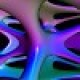# Slides—Differential Forms in $R^n$

A differential $k$-form describes a $k$-dimensional measurement at each point of space. For instance, the area 2-form on a sphere tells us how much each little piece of the sphere should contribute to an integral over the sphere. In this lecture we give some basic facts and definitions about differential $k$-forms, and how to work with them in coordinates. Ultimately differential $k$-forms will pave the way to a general notion of integration, which in turn will be our basic mechanism for turning smooth equations into discrete ones (by integrating over elements of a mesh).## 2 thoughts on “Slides—Differential Forms in $R^n$”

1.Pedroth says:

The link for the slides is not working, I was really looking forward to seeing them 😀

1.Keenan says:

Thanks; now fixed!